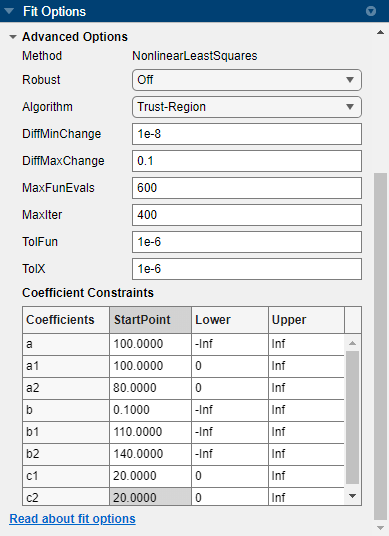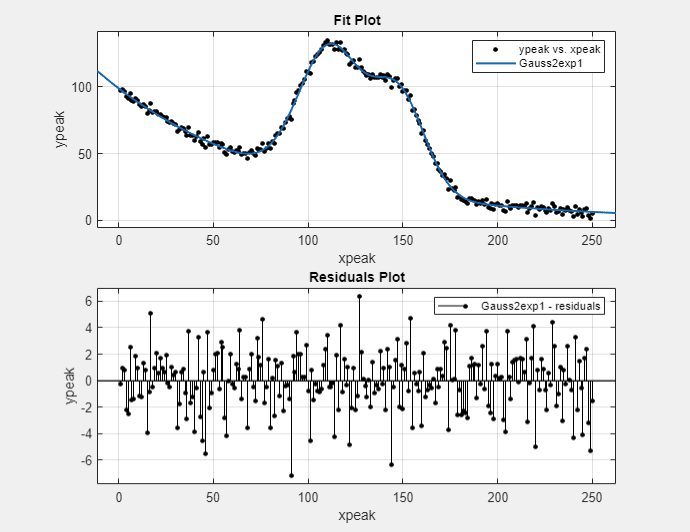Documentation

## Gaussian Fitting with an Exponential Background

This example fits two poorly resolved Gaussian peaks on a decaying exponential background using a general (nonlinear) custom model.

Fit the data using this equation

`$y\left(x\right)=a{e}^{-bx}+{a}_{1}{e}^{-{\left(\frac{x-{b}_{1}}{{c}_{1}}\right)}^{2}}+{a}_{2}{e}^{-{\left(\frac{x-{b}_{2}}{{c}_{2}}\right)}^{2}}$`

where ai are the peak amplitudes, bi are the peak centroids, and ci are related to the peak widths. Because unknown coefficients are part of the exponential function arguments, the equation is nonlinear.

1. Load the data and open the Curve Fitting app:

```load gauss3 cftool```

The workspace contains two new variables:

• `xpeak` is a vector of predictor values.

• `ypeak` is a vector of response values.

2. In the Curve Fitting app, select `xpeak` for X data and `ypeak` for Y data.

3. Enter `Gauss2exp1` for the Fit name.

4. Select `Custom Equation` for the model type.

5. Replace the example text in the equation edit box with these terms:

`a*exp(-b*x)+a1*exp(-((x-b1)/c1)^2)+a2*exp(-((x-b2)/c2)^2)`

The fit is poor (or incomplete) at this point because the starting points are randomly selected and no coefficients have bounds.

6. Specify reasonable coefficient starting points and constraints. Deducing the starting points is particularly easy for the current model because the Gaussian coefficients have a straightforward interpretation and the exponential background is well defined. Additionally, as the peak amplitudes and widths cannot be negative, constrain a1, a2, c1, and c2 to be greater than 0.

1. Click .

2. Change the bound for a1, a2, c1, and c2 to `0`, as the peak amplitudes and widths cannot be negative.

3. Enter start points as shown for the unknown coefficients.

UnknownsStart Point
`a`100
`a1`100
`a2`80
`b`0.1
`b1`110
`b2`140
`c1`20
`c2`20As you change fit options, the Curve Fitting app refits. Press Enter or close the Fit Options dialog box to ensure your last change is applied to the fit.

Following are the fit and residuals.